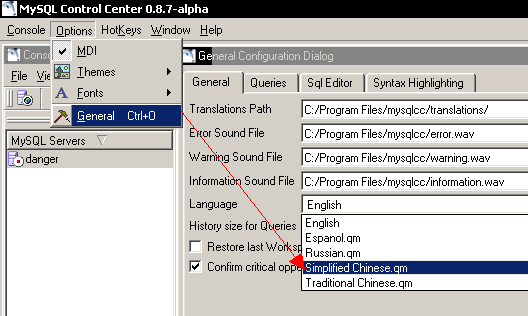```function resize_img()
{
box=document.all("imgbox");
imgall=box.getElementsByTagName("img");
for(n=0;n<imgall.length;n++)
{
if(imgall[n].width>=imgbox.width)
imgall[n].width=imgbox.width;
}
}```

```function resize_img()
{
widarr=document.all("wid");
box=document.all("imgbox");
imgall=box.getElementsByTagName("img");
var widthall=new Array();
for(n=0;n<imgall.length;n++)
{
widarr.value+=imgall[n].width+",";
imgall[n].width=0;}
with(widarr.value)
{
if(charAt(length-1)==",")
newwidth=substring(0,length-1);
widthall=newwidth.split(',')
}
for(n=0;n<imgall.length;n++)
{
if(imgall[n].width==0 &#038;&#038; widthall[n]>imgbox.offsetWidth){
imgall[n].width=imgbox.offsetWidth;}
else if(widthall[n]<imgbox.offsetWidth)
{imgall[n].width=widthall[n]}
}
}```